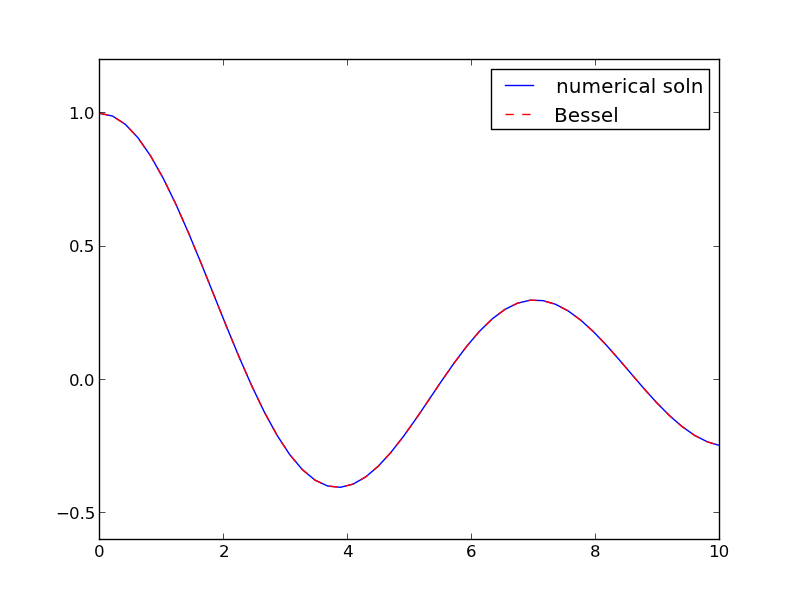## Solving Bessel's Equation numerically

| categories: | tags: | View Comments

Reference Ch 5.5 Kreysig, Advanced Engineering Mathematics, 9th ed.

Bessel's equation $$x^2 y'' + x y' + (x^2 - \nu^2)y=0$$ comes up often in engineering problems such as heat transfer. The solutions to this equation are the Bessel functions. To solve this equation numerically, we must convert it to a system of first order ODEs. This can be done by letting $$z = y'$$ and $$z' = y''$$ and performing the change of variables:

$$y' = z$$

$$z' = \frac{1}{x^2}(-x z - (x^2 - \nu^2) y$$

if we take the case where $$\nu = 0$$, the solution is known to be the Bessel function $$J_0(x)$$, which is represented in Matlab as besselj(0,x). The initial conditions for this problem are: $$y(0) = 1$$ and $$y'(0)=0$$.

There is a problem with our system of ODEs at x=0. Because of the $$1/x^2$$ term, the ODEs are not defined at x=0. If we start very close to zero instead, we avoid the problem.

import numpy as np
from scipy.integrate import odeint
from scipy.special import jn # bessel function
import matplotlib.pyplot as plt

def fbessel(Y, x):
nu = 0.0
y = Y
z = Y

dydx = z
dzdx = 1.0 / x**2 * (-x * z - (x**2 - nu**2) * y)
return [dydx, dzdx]

x0 = 1e-15
y0 = 1
z0 = 0
Y0 = [y0, z0]

xspan = np.linspace(1e-15, 10)
sol = odeint(fbessel, Y0, xspan)

plt.plot(xspan, sol[:,0], label='numerical soln')
plt.plot(xspan, jn(0, xspan), 'r--', label='Bessel')
plt.legend()
plt.savefig('images/bessel.png')You can see the numerical and analytical solutions overlap, indicating they are at least visually the same.

Copyright (C) 2013 by John Kitchin. See the License for information about copying.

org-mode source